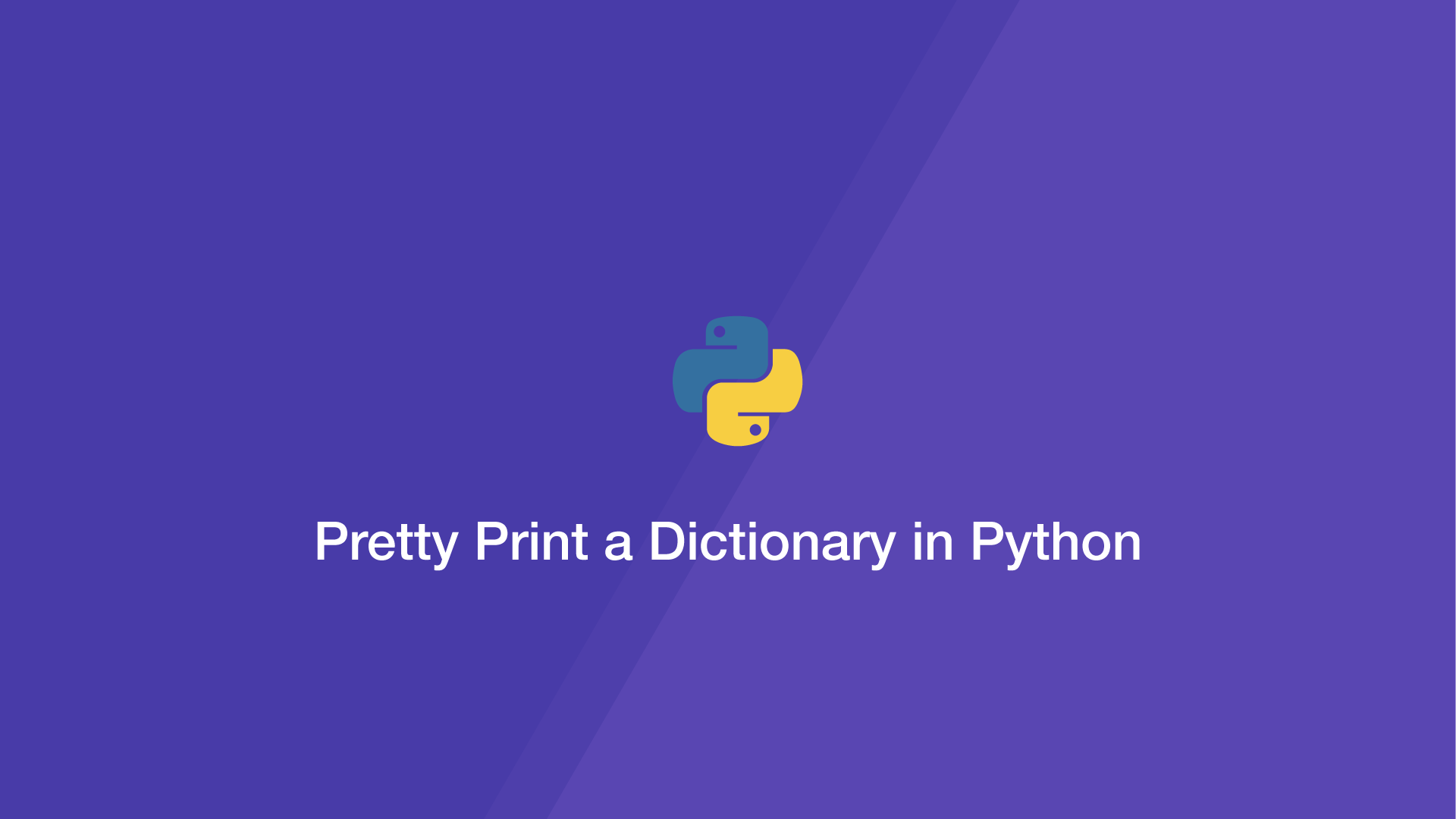# Pretty Print a Dictionary in PythonThis tutorial will cover three different ways of pretty-printing a dictionary in Python.

## Python pprint() Module

The `pprint()` module sorts the data by key value and prints the whole dictionary code. To use it, import the module then call `pprint.print()`, passing the dictionary to pretty print as the first argument.

``````import pprint

items = {'2': 'b', '1': 'a', '3': 'c'}

pprint.pprint(items)
``````
``````{'1': 'a', '2': 'b', '3': 'c'}
``````

If you don't want `pprint()` to sort the dictionary, add the `sort_dicts` parameter and set it to `False` like this:

``````import pprint

items = {'2': 'b', '1': 'a', '3': 'c'}

pprint.pprint(items, sort_dicts=False)
``````
``````{'2': 'b', '1': 'a', '3': 'c'}
``````

If you are working with nested dictionaries `pprint()` is not the ideal solution, as it does not format the output. In those cases `json.dumps()` is more useful.

## Pretty Print with json.dumps()

The Python `json` module contains the `dumps()` function which converts Python objects into JSON formatted data. `json.dumps()` also provides the ability to set the indentation of the JSON and whether to sort the dictionary.

Here is a basic example of `json.dumps()`:

``````import json

items = {'2': 'b', '1': 'a', '3': 'c'}

json.dumps(items)
``````
``````'{"2": "b", "1": "a", "3": "c"}'
``````

Here is another example to sort by key value:

``````import json

items = {'2': 'b', '1': 'a', '3': 'c'}

json.dumps(items, sort_keys=True)
``````
``````'{"1": "a", "2": "b", "3": "c"}'
``````

And another example showing how to add a custom indentation size:

``````import json

items = {'2': 'b', '1': 'a', '3': 'c'}

json.dumps(items, sort_keys=True, indent=4)
``````
``````'{
"1": "a",
"2": "b",
"3": "c"
}'
``````

## Pretty Print with yaml.dump()

It is possible to print a Python dictionary in the YAML format using the `yaml.dump()` function like this:

``````import yaml

items = {'2': 'b', '1': 'a', '3': 'c'}

yaml.dump(items)
``````
``````'1': a
'2': b
'3': c
``````

If you want the cleanest possible printing YAML seems to be the way to go though it works the same as `json.dumps()` in terms of functionality.

#### Related Tutorials### How to Read CSV Files in Python

October 02, 2020### How to Parse JSON Data in Python (Read and Write)

September 18, 2020### How to Calculate Mean, Median, Mode and Range in Python

October 07, 2020### How to Use Dictionaries In Python

September 08, 2020### How to Sort Dictionaries by Value In Python

September 22, 2020October 06, 2020